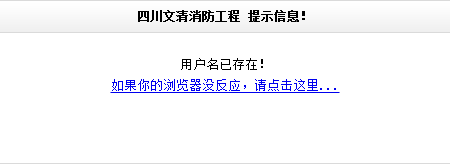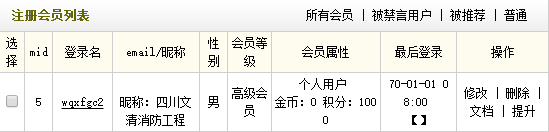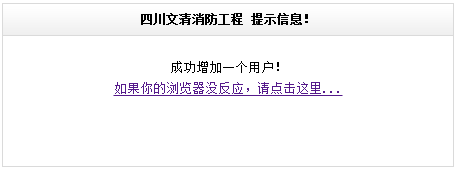1、更新网站时错误

\$innerTmp = \$arcTag->GetInnerText();

\$innerTmp = (\$arcTag=="") ? trim(\$arcTag) : trim(\$arcTag->GetInnerText());
2、网页中图片不显示

function GetOneImgUrl(\$img,\$ftype=1){
if(\$img <> ''){
\$dtp = new DedeTagParse();
if(is_array(\$dtp->CTags)){
foreach(\$dtp->CTags as \$ctag){
if(\$ctag->GetName()=='img'){
\$width = \$ctag->GetAtt('width');
\$height = \$ctag->GetAtt('height');
\$imgurl = trim(\$ctag->GetInnerText());
\$img = '';
if(\$imgurl != ''){
if(\$ftype==1){
\$img .= \$imgurl;
}
else{
\$img .= '<img src="'.\$imgurl.'" width="'.\$width.'" height="'.\$height.'" />';
}
}

}
}
}
\$dtp->Clear();
return \$img;
}
}

[field:bigpic function='GetOneImgUrl(@me,0)'/]  //调用图片的信息，自动调用宽度和高度（已包含Html代码的<img />）
[field:bigpic function='GetOneImgUrl(@me,1)'/]  //只调用图片地址，一般可用于背景图嵌套

{dede:field name='bigpic' function='GetOneImgUrl(@me,1)'/}

3、增加用户时，提示用户名已存在